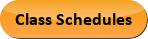Sep 21, 2023
2022-2023 College Catalog
 Select a Catalog 2023-2024 College Catalog 2022-2023 College Catalog [ARCHIVED CATALOG] 2021-2022 College Catalog [ARCHIVED CATALOG] 2020-2021 College Catalog [ARCHIVED CATALOG] 2019-2020 College Catalog [ARCHIVED CATALOG] *** DRAFT 2024-2025 College Catalog DRAFT *** [ARCHIVED CATALOG]
 HELP 2022-2023 College Catalog [ARCHIVED CATALOG] Print-Friendly Page (opens a new window) Add to Portfolio (opens a new window)

# MAT 151 - College Algebra [SUN# MAT 1151]

4 Credits, 4 Contact Hours
4 lecture periods 0 lab periods

Introduction to college-level algebra. Includes functions, exponential and logarithmic functions, linear 2x2 and higher systems, graphing, and calculator use. A graphing calculator is required.

Prerequisite(s): Within the last three years: MAT 095  or MAT 097  or MAT 122 or MAT 122Z or MAT 123 with a grade of C or better, or required score on the Mathematics assessment test.
Information: Credit for only one course will be awarded to students completing MAT 151 and MAT 188 . See course description or advisor to choose your best option. No more than 7 credits may be applied toward graduation from the following list of courses: MAT 151, MAT 182, MAT 187, MAT 188 , and MAT 189 . A graphing calculator is required. See your instructor for details. Access to a scanner required for math classes taken online.
Gen-Ed: Meets AGEC - MATH; Meets CTE - M&S.Course Learning Outcomes
1. Define functions and determine the domain and range. Perform operations on functions.
2. Solve various types of equations and systems.
3. Graph functions and inequalities.
4. Solve problems involving real world applications.

Performance Objectives:
1. Define a function in terms of ordered pairs, graphically, and algebraically.
2. Determine the domain of a function, and determine whether an element is in the range of a function.
3. Use the algebra of functions and composition of functions defined by the modes in objective.
4. Use the definition of a one-to-one function and compute the inverse of a one-to-one function.
5. Define and calculate, exactly and by approximation, zeros and intercepts of functions.
6. Perform basic operations with complex numbers.
7. Find the zeros of polynomial functions by approximation and using simple algebraic methods.
8. Given its zeros and their multiplicities, construct a polynomial function and sketch its graph.
9. Graph rational functions.
10. Solve nonlinear inequalities graphically.
11. Use the properties of exponential functions.
12. Use the concept of inverse functions to develop and work with logarithmic functions.
13. Solve exponential and logarithmic equations.
14. Solve applications, by algebraic means and by approximation, using polynomial, single radical, power, rational, exponential, and logarithmic functions.
15. Solve application problems using linear systems.
16. Use graphing calculators (or other technology).
17. Using technology to model data (linear regression).

Outline:
1. Functions
1. Definition
1. By ordered pairs from table or other sources
2. Graphing
3. Algebraically
4. Piecewise-defined functions
5. Increasing/decreasing functions
6. Even and odd functions
7. Domain and range
1. Determine the domain
2. Determine whether a number is in the range; find the range in other cases.
2. Computations
1. Algebra of functions
2. Composition
3. Find the inverse of a one-to-one function
4. The zeros of a function
2. Polynomial and Rational Functions
1. Computations
1. Identify zeros and y-intercepts
2. Remainder and Factor Theorems
3. Polynomial long division
4. Fundamental Theorem of Algebra
5. Applications of Polynomials
6. Non-linear inequalities (using graphical methods)
7. Complex number systems
2. Second degree polynomials
1. Complete the square to put in a form to identify vertex
2. Applications of maximum/minimum type
3. Rational Functions
1. Use properties of polynomials to analyze rational functions
2. Applications of rational functions
3. Exponential and Logarithmic Functions
1. Properties and relationships
1. Relate exponential and logarithmic as inverse functions
2. Properties of Logarithms
2. Problem solving
1. Use part A to solve exponential and logarithmic equations
2. Formulate and solve applied problems using exponential logarithmic functions.
4. Linear 2 x 2 and Higher Systems
1. Solutions
1. Identify solutions as ordered n-tuples
2. Classify systems as consistent or inconsistent
3. Applications of systems
1. Methods of solution
1. Graphing
1. Determine and graph features of functions and equations in general, and in particular for the types of functions listed in I-III.
1. Intercepts
2. Zeroes
3. Asymptotes
4. Use translations, reflections, and similar operations to obtain a new graph from a given graph.
5. Use graph to interpret and analyze applied problems.
1. Simple radical functions and power functions
2. Calculator Use
1. Numerical calculations and evaluation of functions
2. Graph and analyze functions
3. Other applications such as programs
4. Linear regression
3. Optional Topics
1. Combinatorics
2. The Binomial Theorem
3. Conic sections
4. Systems of equations which include nonlinear equations
5. Systems of linear and/or nonlinear inequalities
6. Mathematical induction
7. Utilizing other types of technology such as spreadsheets
8. Matrices
9. Sequences and Series

Add to Portfolio (opens a new window)